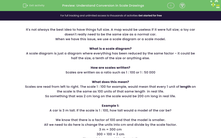# Understand Conversion in Scale Drawings

In this worksheet, students will practise converting between real life and scale lengths.Key stage:  KS 4

Year:  GCSE

GCSE Subjects:   Maths

GCSE Boards:   AQA, Eduqas, Pearson Edexcel, OCR,

Curriculum topic:   Geometry and Measures, Mensuration

Curriculum subtopic:   Mensuration and Calculation Units and Measurement

Difficulty level:#### Worksheet Overview

It's not always the best idea to have things full size. A map would be useless if it were full size; a toy car doesn't really need to be the same size as a normal car.

When we have this issue, we use a scale diagram or a scale model.

What is a scale diagram?

A scale diagram is just a diagram where everything has been reduced by the same factor - it could be half the size, a tenth of the size or anything else.

How are scales written?

Scales are written as a ratio such as 1 : 100 or 1 : 50 000

What does this mean?

Scales are read from left to right. The scale 1 : 100 for example, would mean that every 1 unit of length on the scale is the same as 100 units of that same length  in real life.

So something that was 2 cm long on the scale would be 200 cm long in real life.

Example 1:

A car is 3 m tall. If the scale is 1 : 100, how tall would a model of the car be?

We know that there is a factor of 100 and that the model is smaller.

All we need to do here is change the units into cm and divide by the scale factor.

3 m = 300 cm

300 ÷ 100 = 3 cm

The model car would be 3 cm tall.

Example 2:

A model is made of a 2 m tall man. If the model is 4 cm tall, what is the scale?

The first thing we should notice here is that the units are different, so we need to make them the same.

2 m = 200 cm

Step 2 is to write these numbers as a ratio (remember the model comes first))

4 : 200

Out third and final step is to simplify this ratio. In this example, we can divide both numbers by 4 to give

1 : 50

Now let's have a go at some questions on this.

### What is EdPlace?

We're your National Curriculum aligned online education content provider helping each child succeed in English, maths and science from year 1 to GCSE. With an EdPlace account you’ll be able to track and measure progress, helping each child achieve their best. We build confidence and attainment by personalising each child’s learning at a level that suits them.

Get started••••